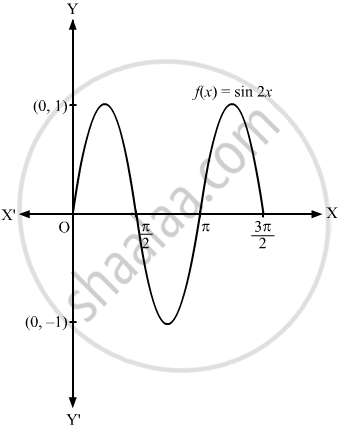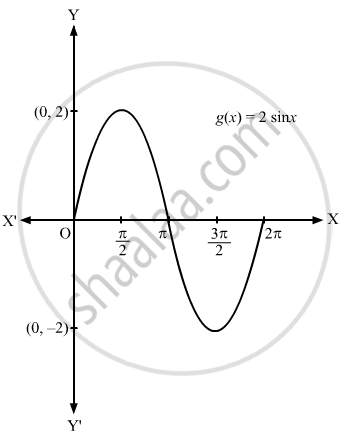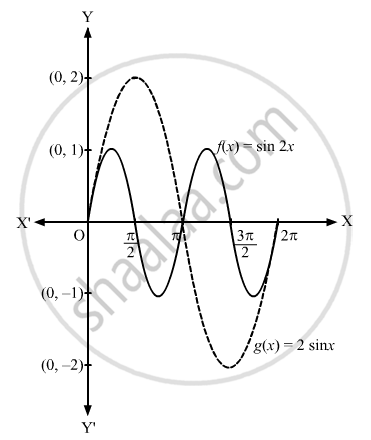Department of Pre-University Education, KarnatakaPUC Karnataka Science Class 11
Advertisement Remove all ads

# Sketch the Graph of the Following Pair of Functions on the Same Axes:F(X) = Sin 2x, G(X) = 2 Sin X - Mathematics

Answer in Brief

Sketch the graph of the following pair of functions on the same axes:
f(x) = sin 2x, g(x) = 2 sin x

Advertisement Remove all ads

#### Solution

f(x) = sin 2x, g(x) = 2 sin x

Clearly, sin 2x and 2 sin x is a periodic function with period π and 2π, respectively.

The graphs of f(x) = sin 2x and g(x) = 2 sin x on different axes are shown below:If these two graphs are drawn on the same axes, then the graph is shown below.Concept: Graphs of Trigonometric Functions
Is there an error in this question or solution?
Advertisement Remove all ads

#### APPEARS IN

RD Sharma Class 11 Mathematics Textbook
Chapter 6 Graphs of Trigonometric Functions
Exercise 6.1 | Q 2.3 | Page 5
Advertisement Remove all ads
Advertisement Remove all ads
Share
Notifications

View all notifications

Forgot password?